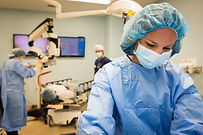## Misuramenti for angulr deformity

#### Correction1. Measure the angle between the axis of the
proximal and distal segments in Cr-Cd
projection. This is the deformity on the frontal
plane. In this example, the angle is 42° - 5° of
physiological valgus = 37° of valgus.2. Measure the angle between the axis of the
proximal and distal segments in ML projection.
This is the deformity on the sagittal plane. In
this example, the angle is 39° - 10° of
physiological procurvatus = 29° of procurvatus.3. Measure the angle between the axis of the
proximal and distal segments in MD projection.
Draw the bisector of the angle: this is the plane of deformity. The hinges should be put on this plane tangent to the cortex.4. Measure the torsional deformity by flexing and extending the carpus, holding the elbow in the sagittal plane. In this example, 75°.5. Draw the hinges' position on the ring using the registered data.6. Set the distal ringfollowing the drawing.7. Set the proximal block up.8. Connect the proximal block with the
distal ring.